## research papersJOURNAL OFSYNCHROTRONRADIATION
ISSN: 1600-5775
Volume 15| Part 5| September 2008| Pages 463-468

## Classification of Fe-bearing species from K-edge XANES data using two-parameter correlation plots

aAdvanced Light Source, Lawrence Berkeley National Laboratory, MS 6-2100, Berkeley, CA 94720, USA, and bSpace Sciences Laboratory, UC Berkeley, Berkeley, CA 94720-7450, USA
*Correspondence e-mail: mamarcus@lbl.gov

(Received 31 March 2008; accepted 16 June 2008; online 19 August 2008)

Solid iron compounds are extremely common in the environment as well as in meteorites and comets. Fe K-edge XANES (X-ray absorption near-edge structure) measurements can be carried out quickly, theoretically allowing one to categorize many areas within a sample or set of samples in a short time. However, interpretation of such data is not straightforward unless one has the appropriate reference spectra, hence a way of classifying an unknown spectrum to a family group (trivalent, divalent, oxide, silicate etc.) is required. Methods of abstracting Fe XANES spectra to produce pairs of variables which, when plotted, cluster in distinct regions depending on the family are presented. For instance, divalent minerals fall in a different region than trivalent minerals, and sulfides in a different region than oxides.

### 1. Introduction

#### 1.1. Motivation

Solid iron compounds are among the most abundant materials in the terrestrial and oceanic environment and in samples from space, such as meteorites and Stardust (Flynn et al., 2006) materials. Iron minerals are not only common, but have important geochemical roles as sorbents and reactants. For instance, goethite and ferrihydrite, which can have large surface areas, sorb various heavy metals (Manceau et al., 2000, 2004; Trivedi et al., 2004; Marcus, Manceau et al., 2004). In another example, Lam et al. (2006) found that some Fe-rich particles in the ocean were composed of silicates, suggesting a continental origin. In addition to these geological and planetary contexts, the chemistry of Fe-bearing materials is important in materials science, archeometry and many other fields. From these examples and many others, it is clear that speciation of Fe-bearing materials can be very useful in a wide variety of fields. Further, the increasing use of microprobe techniques means that in each investigation there may be many spots to speciate on a given sample. The inhomogeneity and complexity of natural materials makes it likely that Fe speciation will vary from spot to spot in a sample. For all these reasons, it is useful to be able to inspect many points in a sample and derive at least a rough speciation for each, to the level of chemical family (sulfide versus oxide, trivalent versus divalent, for instance). The technique of Fe K-edge XANES would seem to be ideally suited for such purposes, being element-selective, sensitive to chemical state, and not requiring much sample preparation. However, such spectra are cryptic, and there is as yet no way to invert' them using theory to derive structure or even valence. Still, on looking at Fe XANES from various phases, it is clear that there are general trends, so that an experienced spectroscopist can distinguish between broad chemical groups.

A number of investigators have worked on empirical ways of classifying Fe XANES spectra by valence, if nothing else. The usual procedure is to look at the pre-edge peaks and measure their energy, amplitude and the ratio of intensities of the (typically) two pre-edge peaks seen in oxides and silicates. Berry et al. (2003), for instance, have worked with Fe-bearing glasses melted and solidified under various oxygen fugacities, so that the average valence varied from 2 to 3, and correlated the valence measured by other techniques with the XANES spectra. This method is highly accurate, but restricted to materials similar in some sense to silicate glass, and also requires excellent data and energy calibration.

#### 1.2. Two-dimensional classification of Fe XANES

We present two methods in which the features of the XANES spectra are reduced to two variables and these variables plotted onto a graph, generating a point for each of many known compounds of various types. In one method the variables are the values of the background-subtracted and normalized XANES signal (normalized to go from 0 to 1 well above the edge) at certain well chosen energies. The energies are chosen so that points corresponding to different chemical families form distinct clusters. This type of plot is similar to the Wilke method (Wilke et al., 2001), except that no attempt at fitting the pre-edge region is made. Instead, the values of the XANES signals at energies in the pre-edge and main-edge regions are used directly. Such plots require very good data and energy calibration, and are best done using the same energy resolution as used for the reference materials. The second method is self-calibrating' in that the zero of energy is taken to be the first inflection point in the pre-edge region, and the coordinates are the energies at which features of the XANES signal occur, relative to this energy zero. In this method we use data at the main rise of the edge instead of just the pre-edge, so that the signal is stronger. These relative-energy' or self-calibrating' methods are thus good when the data are not of as high a quality as one might want or when they come from a source such that the calibration is uncertain. With these two types of plot, i.e. the absolute' (Wilke et al., 2001, 2005) and relative-energy' plots, information can be obtained about the chemical state of an unknown, even if the exact species is not in one's reference database. In a sense, the salient features of the spectra in the large database that we have collected are encoded in the boundaries of various regions of the plots. The aim is that, for instance, an unknown trivalent silicate will plot in a region defined by the trivalent silicates in our database, even if the unknown is not in our database or even in any database.

We emphasize again that these methods are purely empirical. What makes it possible to establish the classification schemes we present here is that we have data from a large and varied collection of Fe species, most taken at the same beamline, and carefully calibrated and processed.

### 2. Methods

#### 2.1. Data acquisition

Most data were acquired at the Advanced Light Source, beamline 10.3.2 (Marcus, MacDowell et al., 2004), which is a microfocus XRF (X-ray fluorescence), XAS (X-ray absorption spectroscopy) and XRD (X-ray diffraction) facility which delivers a beam of size 3 µm × 3 µm to 16 µm × 7 µm (horizontal × vertical). The vertical entrance slits were kept at 20 µm, which yielded an energy resolution limited by the Si (111) monochromator crystals and not the input divergence on the monochromator. Energy calibration was carried out using Fe foil in transmission, with the inflection point taken as 7110.75 eV (Kraft et al., 1996). We used a monochromator glitch at 7263.74 eV as an internal calibrant, having found this glitch to occur at a stable energy. Spectra were recorded from 100 eV below the edge to 300 eV above, with the near-edge and monochromator-glitch region scanned at 0.5 eV intervals or less. To minimize overabsorption and the hole effect (Goulon et al., 1982; Manceau et al., 2002), we performed fluorescence mapping and chose sufficiently small particles on which to record the data that overabsorption was not a concern. We used fluorescence or transmission detection for the XANES measurements, depending on which gave the best results.

Because the synchrotron beam is horizontally polarized, there is significant dichroism in the spectra taken from single-crystalline grains. For many minerals the aforementioned small particles are single-crystalline. Also, the database is intended for use with data acquired under microfocus conditions, so the unknowns to which the data are to be compared may also be dichroic. In order to have at least a rough accounting for polarization, we recorded data on four to nine grains of all non-cubic crystalline materials. For the purposes of this paper, we also constructed approximate powder averages by averaging together the spectra from individual grains.

Dead-time correction, energy calibration with reference to the monochromator glitch, deglitching, pre-edge background subtraction and post-edge normalization were all carried out using LabView-based programs available at the 10.3.2 beamline website (https://xraysweb.lbl.gov/uxas/Beamline/Software/Software.htm ).

Some of our data came from the public database of Newville et al. (1999), a few from the O'Day group (O'Day et al., 2004) and some were provided by people listed in the Acknowledgements. For some of these shared spectra, the energy calibration is known and the spectra could be used in the same way as spectra we recorded ourselves. For some others, the calibration is not known, so they could be used only for the relative-energy plots.1

#### 2.2. Materials

Many of the materials that we used are naturally occurring minerals. Specimens were acquired from Excalibur Minerals or Mineralogical Research Co. Synthetic olivines were obtained from the Natural History Museum, London. Some minerals were obtained from the mineral collection of the Earth and Planetary Sciences Department of the University of California at Berkeley. Minerals were checked for colour and magnetic properties (for those supposed to be magnetic) before use.

Samples were ground with a mortar and pestle and dusted onto Kapton tape, or scratched onto an alumina plate and the resulting streak transferred to tape. As mentioned above, XRF mapping was performed first and grains containing the wrong elements for the nominal mineral were not examined further. Additional verification of species was carried out by performing X-ray diffraction. These precautions were necessary because even the highest-quality specimen often contains inclusions of other phases, and some specimens have proven to be not at all as shown on their labels.

### 3. Absolute-energy plots

We evaluated the use of normalized XANES spectra at fixed energies, as well as their first and second derivatives, to classify minerals. Such an approach has the advantage of linearity, i.e. the values in a mixture of species should be a linear combination of the values for the individual species alone. However, it requires accurate energy calibration, so is not self-calibrating. We defined θ, κ and μ as the normalized XANES absorption values at 7110 eV, 7113 eV and 7117.5 eV. κ′′ is defined as 2κθμ, and is a proxy for the second derivative of the spectrum at 7113 eV. These energies were determined empirically to give the best separation between families in correlation plots of κ versus μ and κ′′ versus μ (Fig. 1). We show only the κ versus μ plot because the κ′′ versus μ plot yields about the same separation between families. In this plot, Fe0 covers silicides (FeSi, FeSi2, Fe3Si) as well as metallic Fe and Fe–Ni alloys (kamacite, awaruite). We see that the Fe2+ and Fe3+ data cleanly segregate.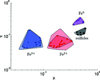Figure 1 Scatterplot of κ versus μ for families of minerals of various oxidation states. The points represent the powder-average spectra from the reference minerals. The darker polygons are the convex hulls surrounding the sets of points corresponding to the different classifications. The outer lighter polygons surround the points corresponding to spectra from individual grains, showing the effects of dichroism and polarization.

Overabsorption (Manceau et al., 2002) causes distortions of the XANES spectrum, thus shifting points on the plots. In general, small signal values increase and large ones decrease. Thus, one can find an apparent admixture of Fe2+ in a pure Fe3+ spectrum because the signal rises more quickly at lower energies than it would otherwise. However, XANES-classification methods which use only the pre-edge are almost immune to this effect because the absorbance is small at the pre-edge.

### 4. Relative-energy plots

#### 4.1. Methodology

In order to define a zero of energy that is independent of calibration errors, we differentiate the data and take the first maximum of the derivative in the pre-edge region, near 7110–7115 eV. In some cases we smooth the data using an adjustable tensioned spline, using the minimum amount of smoothing required to obtain a consistent derivative. We then shift the abscissa scale so that the inflection point thus located is at 0 eV. Next, we evaluate the following parameters for each spectrum:

E25,50,75,100, the energy at which the signal first reaches 25%, 50%, 75% and 100% of its post-edge value;

Emax, the energy at which the signal reaches its first maximum after the pre-edge region;

S−1 = [d(XANES)/dE|E=E50]−1, this inverse slope at the half-jump point has dimensions of energy, as do the other measures;

Y10,15,20,25, the values of the normalized XANES curves at 10, 15, 20 and 25 eV.

From this menu of values, we attempted to devise combinations which would effectively classify the species into chemical groups (Fe2+ oxides including silicates, Fe3+ oxides, mixed-valent oxides, sulfides, metal and silicides), and, within the oxide group, allow one to estimate the average valence. For the classification scheme we plotted every combination of two measures, and for the estimation method we fit the valence as a function of up to three of the variables with up to four parameters, V = f(x,y,z;params) where V is the average valence (from the nominal stoichiometry), x,y,z represent any three of the ten values defined above, and params is a set of up to four parameters.

In order to improve the reliability of fitting of the mixed-valent oxides, of which we have few examples, we synthesized spectra consisting of linear combinations of those of Fe2+ and Fe3+ compounds, assigning them average valences according to the weights given the constituent spectra. Note that the locus of points of such a mixture, going from one end-member to the other, is not a straight line as it is in the absolute-energy plots. This is because the values used may be the energies at which the signals attain specified values, rather than the signals at fixed energies, and because the energy origin moves with composition.

#### 4.2. Classification

A good classification scheme, which is also good for fitting with two variables, is to use Emax and E50 as axes. The resulting plot is shown in Fig. 2. The various symbols represent reference compounds in the various groups. Here, we lump the metals [body-centred-cubic (b.c.c.) Fe, awaruite (meteoritic FeNi3 ordered face-centred-cubic solid solution), kamacite (meteoritic b.c.c. Fe:Ni alloy), Fe-based metallic glass, Fe silicides and Fe carbides] into one group. Sulfides are defined as another group, and the rest are oxides and silicates. Note that the plot nicely separates out all the groups, except that FeSi2 and FeSi plot with the sulfides, and a few of the Fe2+ oxides plot with the mixed-valent group. It may be relevant that FeSi2 and FeSi, which plot with the sulfides, are semiconductors (McKinty et al., 2000; Ouyang et al., 2006) as the sulfides are, whereas Fe3Si, which plots with the metals, is a metal (Muir et al., 1982). Some other combinations of variables work almost as well as this one, but none work better. In particular, the combination of E25 and Y10 (Fig. 3) yields a very clear separation of oxides from sulfides and metals, but does not separate divalent from trivalent oxides as well as the scheme using Emax and E50 does.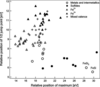Figure 2 Classification plot using two relative-energy variables, Emax and E50. Divalent and trivalent oxides (including silicates) are shown by open and closed triangles, respectively. Mixed-valent oxides are plotted as crosses, metals and intermetallics as open circles, and sulfides as closed circles.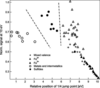Figure 3 Alternate classification plot using two different relative-energy variables, E25 and Y10.

#### 4.3. Fitting

We tried the following types of fits: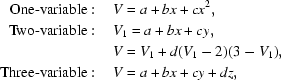where a, b, c and d are parameters. In the two-variable fit, the term on the second line allows the contours of constant valence on the plot to be unequally spaced. This term is zero at either end of the oxide-valence scale and non-zero in the middle, so it shifts the contours in the mixed-valence region but not at the ends. It turns out that for the best-fit combination (Emax versus E50) the value of d is indistinguishable from zero, but the fits for other combinations do take advantage of the d term. The results of best one-, two- and three-variable fits are shown in Fig. 4and the parameter values are listed in Table 1. For each number of variables, the fit to the best combination (lowest mean-square error) is shown. On the abscissa is the true valence of the reference material, and on the ordinate is the error. Note that the best two-variable combination is the same (Emax and E50) as is used in the classification plot.

 Table 1Fit parameters for one-, two- and three-variable fits
Variables a b c d RMS error
Y10 4.1425 −2.2737 1.9583   0.2090
Emax, E50 −1.0660 −0.2642 0.0574 0 0.1959
E25, E50, Y20 −1.4828 −0.2932 0.5260 0.8236 0.1817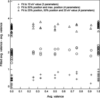Figure 4 Fit errors for the one-, two- and three-variable fits characterized in Table 1. Although the RMS values are not very different, note that the three-variable fit (triangles) does visibly better at the Fe3+ end than the two-variable fit (circles) or the one-variable fit (crosses). The ordinates are shifted upwards by 0.5, 2 and 3.5 units for clarity.

#### 4.4. Mixtures, polarization and overabsorption

In contrast to the case of absolute-energy plots, the locus of points corresponding to mixtures of end-members is not a straight line connecting the end-member points. Some examples of this effect are shown in Fig. 5. As mentioned above, the reasons for this effect involve the movement of the energy origin as one goes from one end-member to another, and the fact that what are plotted are not signal values but the energies at which the signal attains certain values.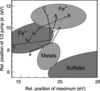Figure 5 Trajectories of points in Emax − E50 plots for simulated mixtures of: a–A: basalt glass–6-line ferrihydrite; b–B: fayalite (Fe2+SiO4)–hematite (Fe3+2O3); c–C: hercynite (Fe2+Al2O4)–goethite (FeOOH). Data are tabulated at fractions of the Fe3+ component (capital letters) of 0, 0.25, 0.5, 0.75 and 1.

Similarly, the polarization effect may be described as the sample appearing to be an orientation-dependent mixture of `end-members'. Fig. 6shows examples of the EmaxE50 plots for individual grains of several minerals at various orientations, and Fig. 7shows the same thing but in an E25Y10 plot. We see that polarization effects can cause mis-classification in the EmaxE50 plot, whereas the E25Y10 plot is more robust. It may be that the position of the maximum varies with polarization because there are typically several maxima, so that one or another becomes most prominent as the orientation changes. On the other hand, the absolute-energy method is quite robust with respect to polarization effects, as seen in Fig. 1, in which the lighter-coloured polygons show the regions occupied by points corresponding to single grains as well as powder averages. We see that the polarization effect does not cause any mis-classification in absolute-energy plots as opposed to the EmaxE50 plot.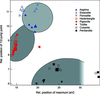Figure 6 Plot of Emax − E50 coordinates for individual grains showing the effect of polarization. The larger symbols represent the average spectrum (average of all grains) used in the other plots in this paper. Red, blue and black symbols represent divalent, trivalent and sulfide compounds, respectively. The compounds are aegirine (closed triangles), esseneite (open triangles), ferrosilite (open triangles, point down), hedenbergite (open squares), pigeonite (closed circles), troilite (closed triangles, point down), cubanite (open circles) and pentlandite (closed squares). Grey regions are the boundaries of the trivalent, divalent and sulfide regions shown in Fig. 2. The sulfide region fades off to the right because we have no data for powders in which Emax > 30 eV.Figure 7 Plot of E25 − Y10 coordinates for individual grains showing the effect of polarization. The details are the same as those in Fig. 6.

The overabsorption effect does not shift the positions of extrema, but does make E50 decrease, thus moving the point corresponding to a given Fe3+ spectrum in an EmaxE50 plot towards the Fe2+ area. Similarly, in the E25Y10 plot, Fe3+ points would move upward and to the left to join the Fe2+ cloud. We see that, like absolute-energy plots, relative-energy plots are vulnerable to overabsorption.

### 5. Conclusions

If the energy calibration and the data are good enough, then absolute-energy plots provide a useful method for the classification of unknown Fe-containing species into rough chemical groups. It is also possible to derive average valences for mixed-valent oxide species. This method is suitable for surveying many particles in samples for which all relevant reference spectra may not be available.

If the data are not well calibrated or the pre-edge signal not strong enough for accurate characterization by the absolute-energy method, then relative-energy plots can still be used for classification and valence measurement, albeit with reduced reliability. Of the two relative-energy plots presented, the Emax − E50 plot provides somewhat more accuracy in measuring the average valence in oxide-type materials, while the E25Y10 plot is better for overall classification by type. In general, both absolute- and relative-energy plots represent methods of encoding the most significant features of a large database into simple plots. It may be that more sophisticated recognition methods, such as those involving artificial neural networks, could perform even better.

It would be interesting to try to apply similar methods to other polyvalent elements such as Co, Mn and Cu. The case of Mn may be especially challenging since this element occurs in three common valences in nature, not counting zero.

### Footnotes

1The Fe K-edge XANES data are available from the IUCr electronic archives (Reference: KV5050 ). Services for accessing these data are described at the back of the journal.

### Acknowledgements

The operations of the Advanced Light Source at Lawrence Berkeley National Laboratory are supported by the Director, Office of Science, Office of Basic Energy Sciences, US Department of Energy under contract number DOE-AC02-05CH11231. We gratefully acknowledge the use of spectra and/or samples from members of the Fendorf and O'Day groups (S. Benner, D. Bond, Th. Borch, B. Bostick, S. Fendorf, C. Hansel, P. Nico, P. O'Day, N. Rivera) as well as C. S. Chan, M. Heuer, A. Kearsley, C. S. Kim, M. Newville, P. Nico, K. Ross, C. Santelli, B. M. Toner, and G. A. Waychunas.

### ReferencesJOURNAL OFSYNCHROTRONRADIATION